# Convert RGB Image to Grayscale by Using a Neighborhood Processing Subsystem Block

This example shows how to convert an RGB image to grayscale by using a Neighborhood Processing Subsystem block. Converting images to grayscale is an important step in image processing tasks such as corner detection. This example implements grayscale conversion using both the mean and luminosity methods, which weigh the red, green, and blue color channels differently. The luminosity method accounts for the different sensitivities that human eyes have for these colors, and the mean method does not.

### Inspect Model

1. Open the model.

```mdl = 'RGBToGrayscaleNeighborhoodExample'; open_system(mdl);```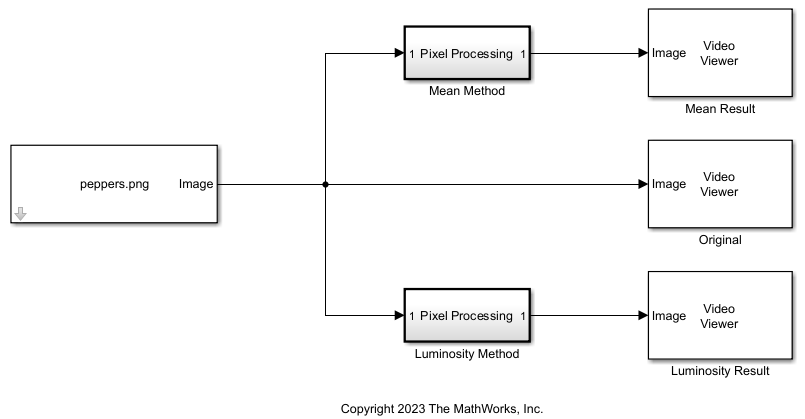The model imports an image by using an Image From File block from Computer Vision Toolbox. The model uses two Neighborhood Processing Subsystem blocks to convert the image to grayscale in two different ways. Each Neighborhood Processing Subsystem block uses the `Valid` option for the Padding option parameter. The `Valid` option configures the Neighborhood Processing Subsystem block to output a matrix that is smaller than the input matrix by excluding elements whose neighborhoods extend beyond the input matrix. By using a Neighborhood size parameter of `[1 1 3]` and the `Valid` option, each Neighborhood Processing Subsystem block converts its RGB input to a single-channel output with the same horizontal and vertical dimensions as the input image.

The model displays the original image and each output image using Video Viewer blocks from Computer Vision Toolbox.

2. Open the `MeanRGB2Gray` subsystem.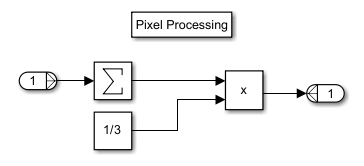The subsystem uses a Sum of Elements block to add the three channel values together, then multiplies the sum by `1/3` to calculate the mean brightness value of the pixel.

3. Open the `LuminosityMethodRGB2Gray` subsystem.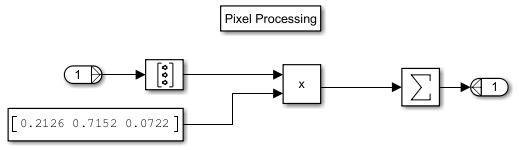The subsystem calculates a weighted mean to account for the different sensitivities human eyes have for red, blue, and green light by:

1. Converting the 1-by-1-by-3 neighborhood to a 3-by-1 column vector by using a Reshape block

2. Multiplying the 3-by-1 column vector by the row vector `[0.2126 0.7152 0.0722]`

3. Passing the product through a Sum of Elements block

### Simulate Model and View Results

Simulate the model.

`sim(mdl);`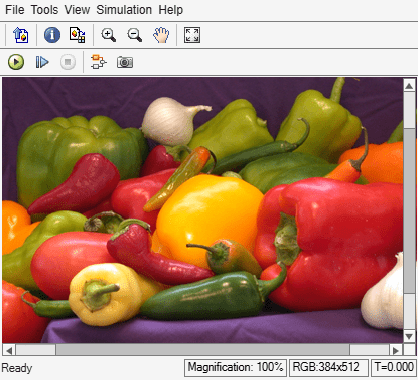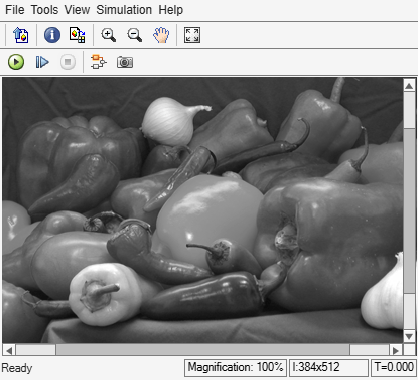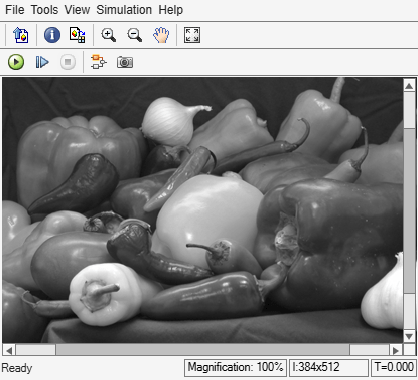Each Neighborhood Processing Subsystem block produces a single-channel grayscale version of the three-channel RGB input image.